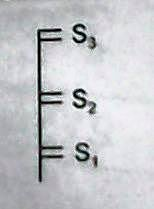# Can you calculate average speed?$S_{1}, S_{2}$ & $S_{3}$ are the different sizes of windows 1, 2 & 3 respectively, placed in a vertical plane. A particle is thrown up in that vertical plane. Average speed of the particle passing the windows may be equal if:

(Assume that the speed of the particle is sufficient so that it crosses all the windows.)

Try more from my set Classical Mechanics Problems.

×